GFG App
Open AppBrowser
Continue

# Difference Between Electric Potential and Potential Difference

The flow of electric charges is known as electricity, and it is responsible for producing electric current. An important word associated with electricity is electric potential. A potential difference is required to create the flow of electrons and hence, produce electricity. Before understanding the difference between electric potential and potential difference, let’s first understand the electric potential and potential difference in detail.

## Electric Potential

Electric potential is the work done per unit charge in order to bring that charge from infinity to a point in the electrostatic field against the field force. Electric Potential is also referred to as Voltage drop. The SI unit for Electric Potential or Electric Potential difference is Voltage or Volts. Electric potential is a scalar quantity.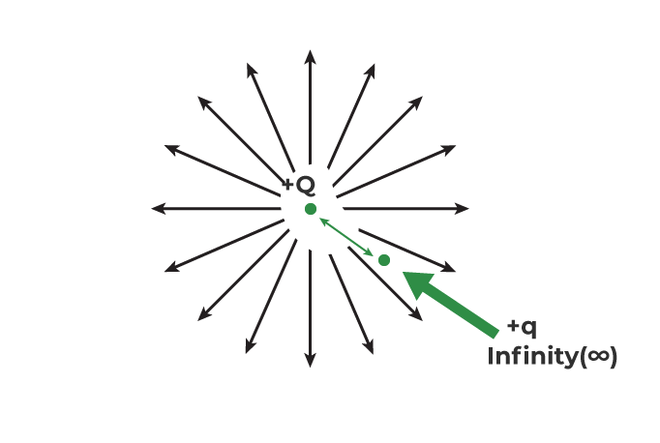In the above figure, +Q is the charge creating an electric field, and the task is to bring a unit charge (+q) from infinity (anywhere outside the electric field) to a point inside the electric field against the field. Since the electrostatic force produced by the electric field will be against the unit charge, some work is required by the unit charge in order to move from either infinity to somewhere in the field or from one point to another in the field.

• If the work is done to move the charge from infinity to point X, it will be called an Electric potential at X (Vx).
• If the work is done to move the charge from infinity to point Y, It will be called an Electric potential at Y (Vy).
• If the work is done to move the charge from X to Y, it will be called the potential difference between x and y (Vxy).

### Electric Potential Formula

Electric Potential/Voltage = Work Done/Unit Charge

### SI unit for Electric Potential

• V = W/q = Joules/Coulomb = Volts

Therefore, the SI unit for Electric Potential is Volts or Voltage.

• 1 Volt = 1 Joule/1 Coulomb

1 Volt can be defined as 1 joule of work done in order to move 1 coulomb of charge

## Electric Potential Difference

Electric potential difference is also known as voltage. The electric potential difference is the work done per unit charge to move a unit charge from one point to another in an electric field. Electric potential difference is usually referred to as a Voltage difference. Imagine a ball sitting at some height, will there be some energy in the ball? Yes, the energy is called Potential energy, and if the ball is dropped from a point A to B height, the ball will always fall from higher gravitational potential to lower, then there will be a difference in both energies. The electrical potential difference is analogical to this concept. The energy possessed by Electric charges is known as electrical energy. A charge with higher potential will have more potential energy, and a charge with lesser potential will have less potential energy. The current always moves from higher potential to lower potential. The difference in these energies per unit charge is known as the electric potential difference. In order to create electricity and the flow of current, a potential difference is always required, which is maintained by a battery or a cell.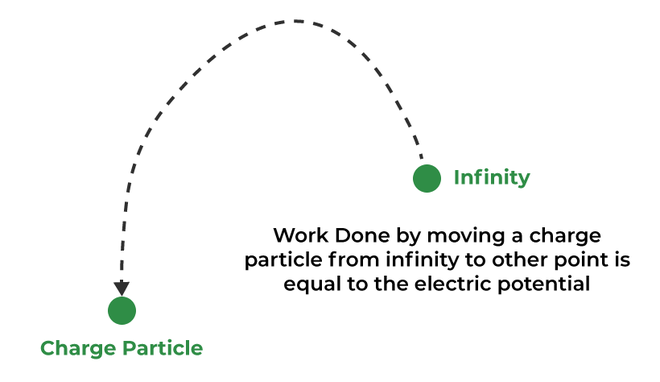The longer side represents the Higher potential (+ve terminal), and the shorter side represents the Lower potential (-ve terminal). The Electric potential difference is measured by a Voltmeter which is applied parallel to the Instrument whose Voltage is to be measured.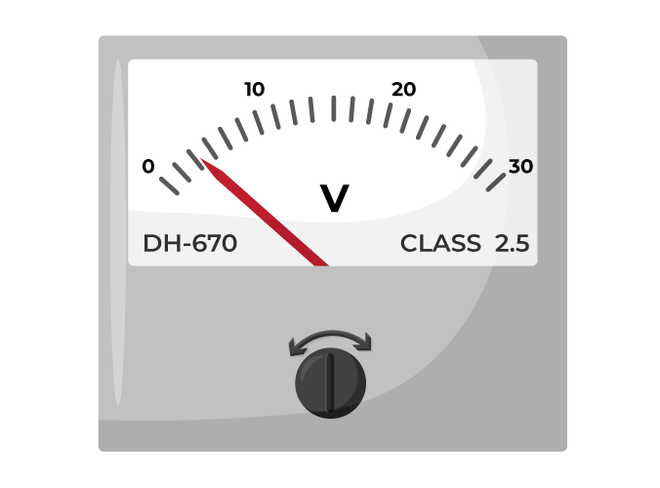### Electric Potential Difference Formula

Vxy= Vx – Vy = [Wx – Wy]/q

### SI Unit of Electrical Potential Difference

The SI Unit of Electrical potential difference is the same as the electric potential, i.e, Voltage or Volts.

Note: Why a unit charge is taken to explain the concept of Electric Potential?

A small unit charge will have very small electric field which will neither affect not distort the field produced by the bigger charge and hence, the concept can be explained easily.

## Ohm’s Law

According to Ohm’s Law, The current flowing through a conductor is directly proportional to the voltage, provided the conductivity and temperature remain constant. While removing the proportionality, a new constant is introduced known as the Resistance.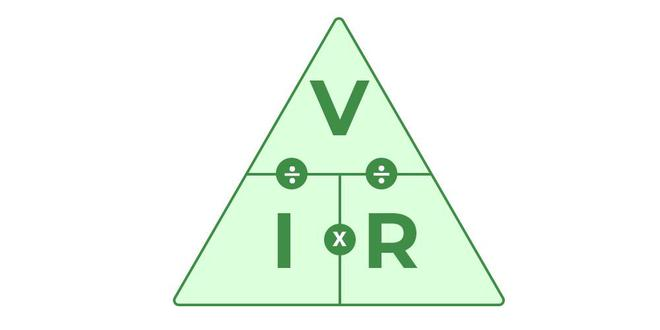V ∝ I

V = IR

Where,

• V = Voltage across the conductor
• I = Current flowing in the wire
• R = Resistance offered by the conductor in ohms

## Solved Examples on Difference Between Electric Potential and Potential Difference

Question 1: A charge of 10mC is moved from infinity to point A in the Electric field. The work done in this process is 20 Joules. What is the Potential difference?

Solution: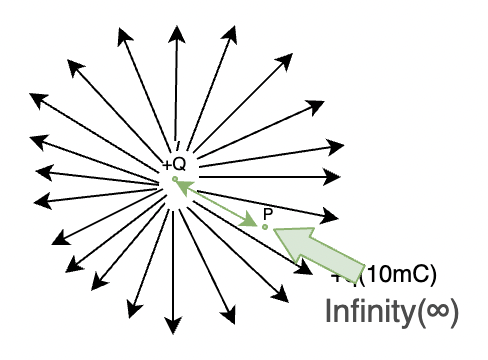V= W/q

W= 20 joules

q= 10mC

V= 20/10×10-3= 2kV

Therefore, the potential developed is 2000volts.

Question 2: A Charge of 50mC is moved from one point to another (from A to B). The voltage at A is 50kV, and the Voltage at B is 30kV, Find the Work done by the charge.

Solution:

VAB = VA– VB = 50kV – 30kV = 20kV

Work done = V× q

= 20kV × 50mC

= 100 Joules

Question 3: What are the SI units of these following quantities: Energy, Potential Difference, Charge, Resistance

The SI Units of the above-mentioned quantities:

Energy⇢ Joules

Potential Difference⇢ Voltage

Charge⇢ Coulomb

Resistance⇢ Ohms

Question 4: Find the current through the circuit when the voltage across the terminal is 30V and the resistance offered by the conductor is 10ohm.

Solution:

According to ohms Law,

V= IR

30V = I × 10ohm

I= 3 Amperes

Therefore, the current through the circuit is 3 amperes.

## FAQs on the Difference Between Electric Potential and Potential Difference

Question 1: What is electric potential?

Electric potential is defined as the work done to bring a unit positive charge from infinity to that point in the electric field. The SI unit of electric potential is volts.

Question 2: What is the potential difference?

Potential difference is the amount of work required to bring a unit charge from one point in an electric field to the other point. The SI unit of potential difference is volts.

Question 3: What is the difference between Electric Potential and Electric Potential difference.

Electric Potential is the work done per unit charge in order to bring the charge from infinity to a point in electric field while Electric potential difference is the Potential developed while moving a charge from one point to another in the field itself.

The SI Unit of both electric potential and electric potential difference is Volts/ Voltage.

Question 4: What happens when the battery is in connection with the circuit?

When the battery is in connection with the circuit, a potential difference is created at the ends of the conductor. The potential difference is responsible for the formation of an electric field throughout the conductor, and hence the current starts to flow from high potential to low potential.

Question 5: What is the difference between EMF and potential difference?